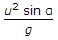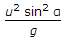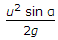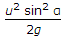# Civil Engineering - Applied Mechanics - Discussion

### Discussion :: Applied Mechanics - Section 2 (Q.No.2)

2.

If α and u are the angle of projection and initial velocity of a projectile respectively, the horizontal range of the projectile, is

 [A].[B].[C].[D].Explanation:

No answer description available for this question.

 Rakesh said: (Mar 15, 2014) R = horizontal range = u^2 sin 2#/g.

 Kaushik said: (Jul 5, 2016) Answer should be U^2sin(2a)/g As range will be horizontal component of velocity i.e Ux multiply by t(time)____ Ux t= Usin(a)*t now for finding t let us consider vertical motion of proectile So, S = ut + 0.5 at^2. 0 = Uy *t - 0.5 * a * t^2 (put Uy as Usin(a)). Solving this we get t = 2Usin(a)/g. Hence range R = Ux * t = Ucos(a) * 2 * Usin(a)/g. Hence R = U^2sin(2a)/g.

 Parv said: (Jul 17, 2016) All options are wrong. It will be: = U^2 SIN2 * ANGLE/G.

 Mandar said: (Nov 16, 2016) Agree @Parv.

 Chhaya said: (Apr 4, 2017) I too Agree @Parv.

 Anshu Singh said: (Jun 30, 2017) No, Given option is correct. It should be v^2sin2θ/g.

 Kishan Kumar said: (Oct 1, 2018) Horizontal range of the projectile, is u2sin (a)/g. Similarly, Toataltime of flight 2usin(a)/g.

 Ajay said: (May 1, 2019) U^2sin2α ÷ 2g this is the correct answer.

 Aswathy said: (Dec 17, 2019) Horizontal range= (u^2sin(2\$))/g Maximum ht=(u^2sin^2(\$))/2g Time to reach maximum ht=(usin(\$))/g Time of flight=(2usin(\$))/g

 Shubham Siddharth Nayak said: (Nov 21, 2020) Maximum horizontal range occurs at 45 degrees. = u sqr/g.